Question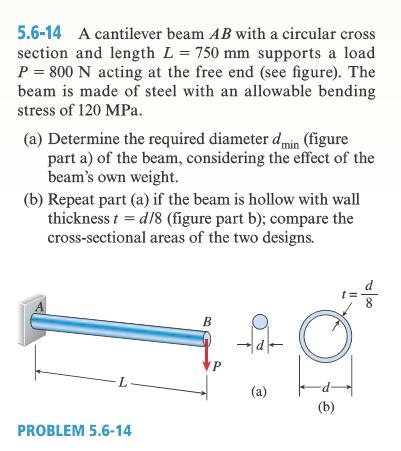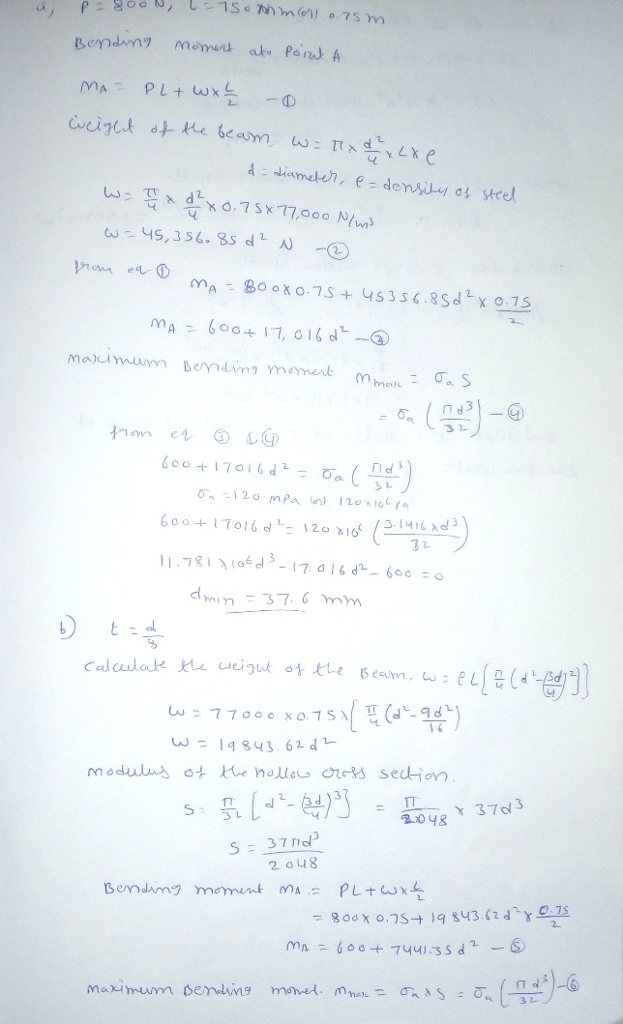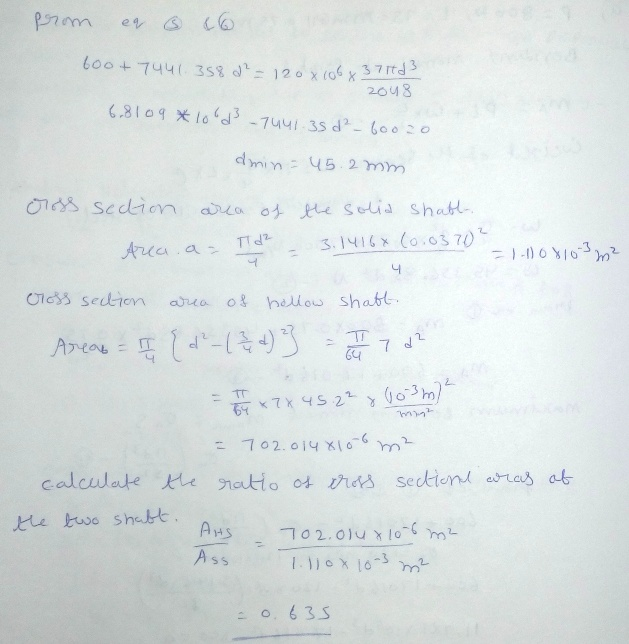#### Earn Coins

Coins can be redeemed for fabulous gifts.

Similar Homework Help Questions
• ### 3. A beam with a hollow circular cross section of outer diameter D and inner diameter...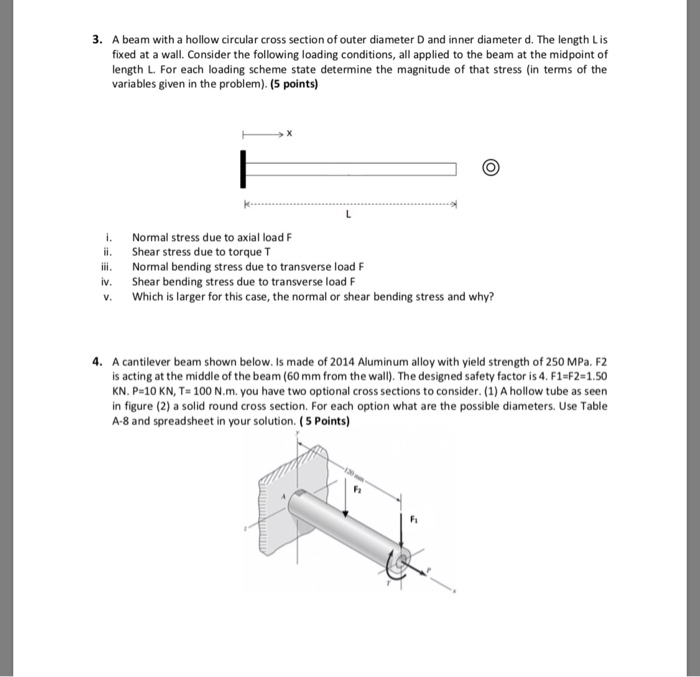3. A beam with a hollow circular cross section of outer diameter D and inner diameter d. The length Lis fixed at a wall. Consider the following loading conditions, all applied to the beam at the midpoint of length L. For each loading scheme state determine the magnitude of that stress in terms of the variables given in the problem). (5 points) i. ii. iii. iv. V. Normal stress due to axial load F Shear stress due to torque T...

• ### A Problem 5 A tapered cantilever beam AB of length L has square cross sections and...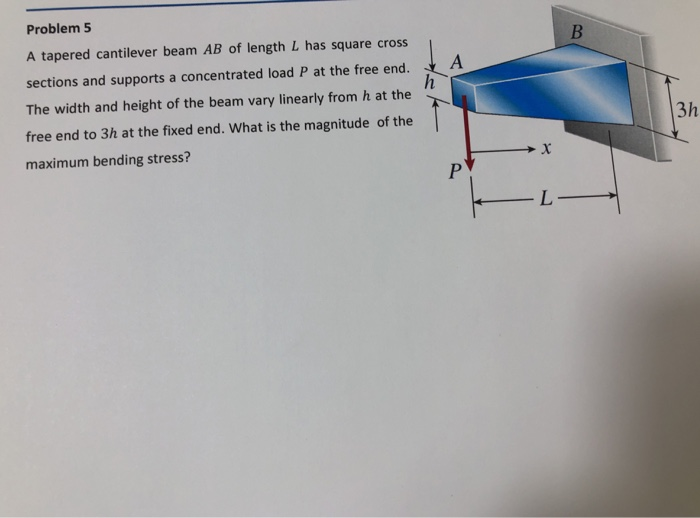A Problem 5 A tapered cantilever beam AB of length L has square cross sections and supports a concentrated load P at the free end. The width and height of the beam vary linearly from h at the free end to 3h at the fixed end. What is the magnitude of the maximum bending stress?

• ### A cantilever beam supports the loads shown. The cross-sectional dimensions of the shape are also shown....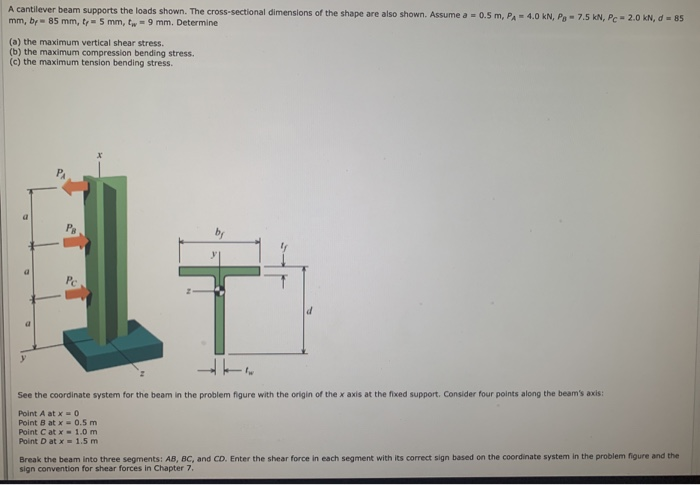A cantilever beam supports the loads shown. The cross-sectional dimensions of the shape are also shown. Assume mm, by - 85 mm, 5 mm, 9 mm. Determine - 0.5 m, P. - 4.0 kN, Pg - 7.5 kN, Pe-2.0 kN, -85 (a) the maximum vertical shear stress. (b) the maximum compression bending stress. (c) the maximum tension bending stress. See the coordinate system for the beam in the problem figure with the origin of the x axis at the feed...

• ### A cantilever wood beam with a span of L = 21 ft supports a linearly distributed...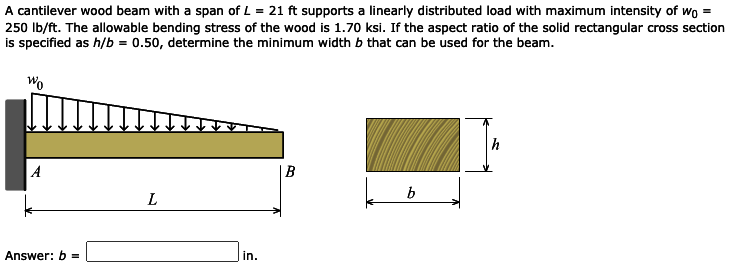A cantilever wood beam with a span of L = 21 ft supports a linearly distributed load with maximum intensity of w0 = 250 lb/ft. The allowable bending stress of the wood is 1.70 ksi. If the aspect ratio of the solid rectangular cross section is specified as h/b = 0.50, determine the minimum width b that can be used for the beam. A cantilever wood beam with a span of L = 21 ft supports a linearly distributed load...

• ### 1.2 (20 Marks) A beam of rectangular cross section (width b and height h) supports a...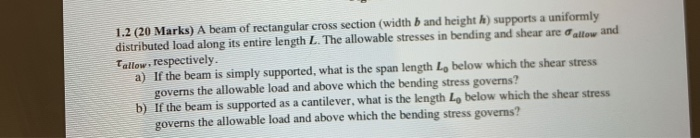1.2 (20 Marks) A beam of rectangular cross section (width b and height h) supports a uniformly distributed load along its entire length L. The allowable stresses in bending and shear are all and Tallow, respectively. a) If the beam is simply supported, what is the span length Lo below which the shear stress governs the allowable load and above which the bending stress governs? b) If the beam is supported as a cantilever, what is the length Lo below...

• ### A cantilever beam supports the loads shown. The cross-sectional dimensions of the shape are also shown....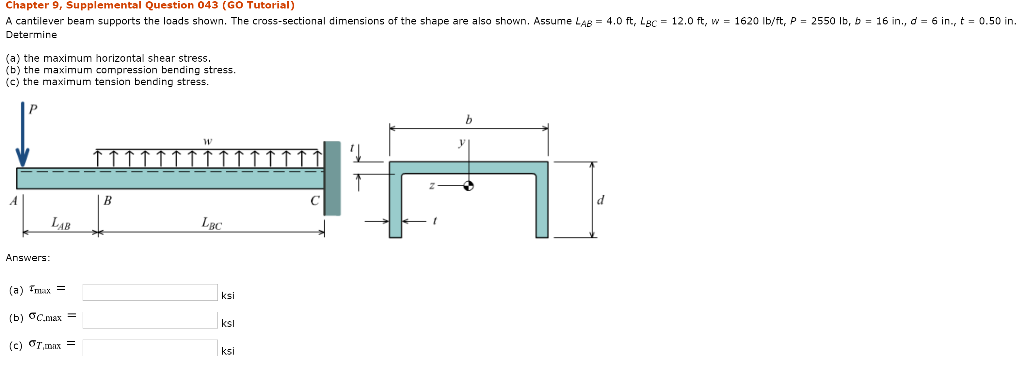A cantilever beam supports the loads shown. The cross-sectional dimensions of the shape are also shown. Assume LAB = 4.0 ft, LBC = 12.0 ft, w = 1620 lb/ft, P = 2550 lb, b = 16 in., d = 6 in., t = 0.50 in. Determine (a) the maximum horizontal shear stress. (b) the maximum compression bending stress. (c) the maximum tension bending stress. Chapter 9, Supplemental Question 043 (GO Tutorial) A cantilever beam supports the loads shown. The cross...

• ### Q1 A cantilever steel beam of length L = 7.5 m carries both a uniformly distributed...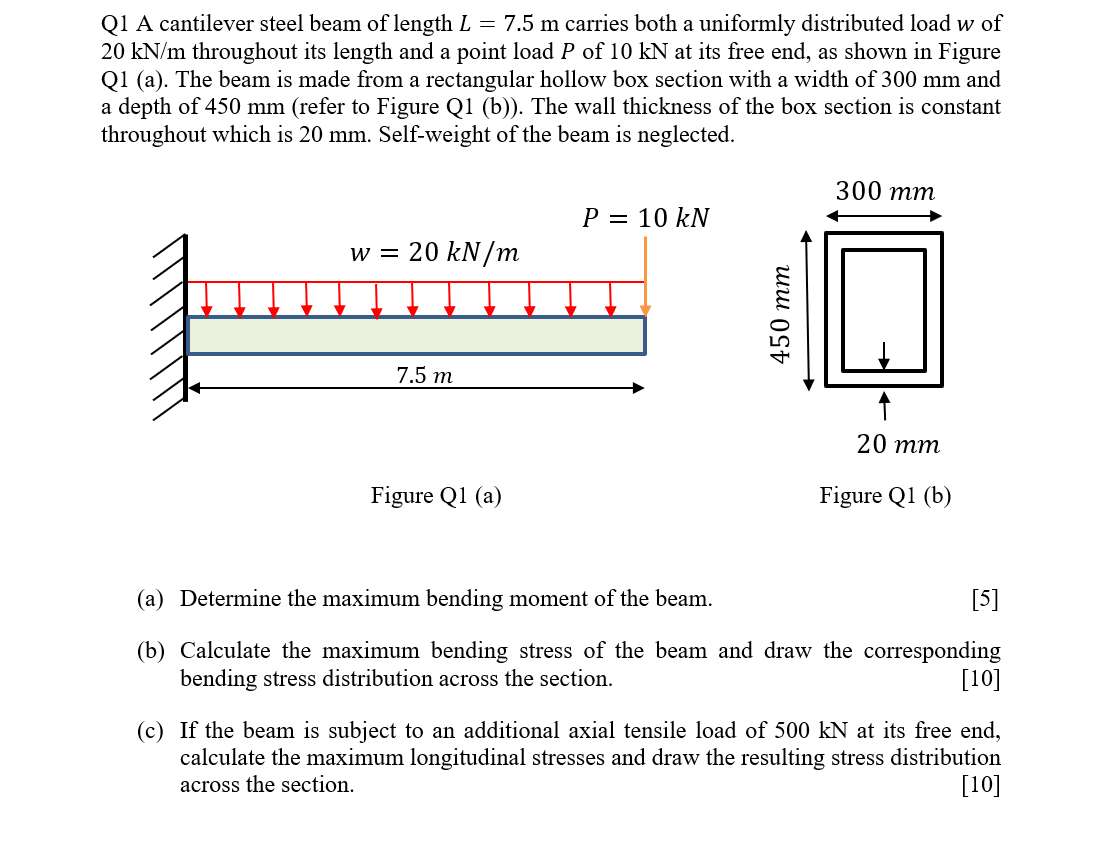Q1 A cantilever steel beam of length L = 7.5 m carries both a uniformly distributed load w of 20 kN/m throughout its length and a point load P of 10 kN at its free end, as shown in Figure Q1 (a). The beam is made from a rectangular hollow box section with a width of 300 mm and a depth of 450 mm (refer to Figure Q1 (b)). The wall thickness of the box section is constant throughout which...

• ### 4. (20 pt) A wood beam AB on simple supports with span length equal to 9...

4. (20 pt) A wood beam AB on simple supports with span length equal to 9 ft is subjected to a uniform load of intensity 120 lb/ft acting along the entire length of the beam and a concentrated load P acting at a point 3 ft from the right-hand support (see figure). The allowable stresses in bending and shear, respectively, are 2500 psi and 150 psi. What is the allowable P? (Note: The width and height of the cross section...

• ### A 49 in long simply supported hollow circular steel tube has a 1.68 in OD and...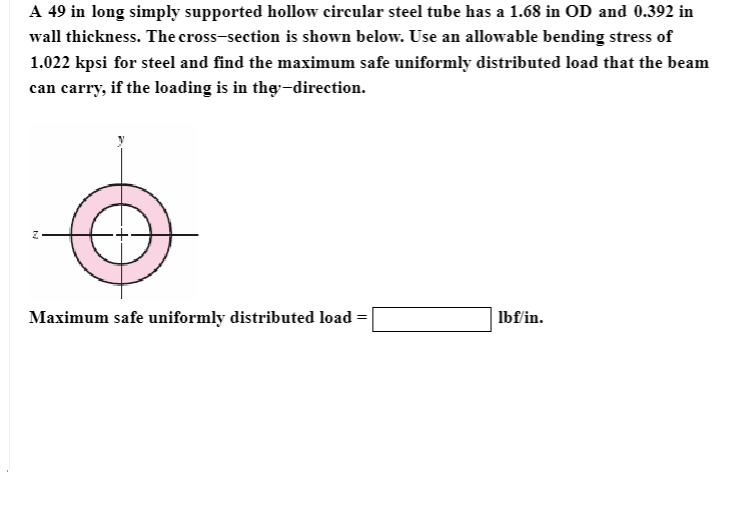A 49 in long simply supported hollow circular steel tube has a 1.68 in OD and 0.392 in wall thickness. The cross-section is shown below. Use an allowable bending stress of 1.022 kpsi for steel and find the maximum safe uniformly distributed load that the beam can carry, if the loading is in the direction. Maximum safe uniformly distributed load- lbf/in.

• ### QI A cantilever steel beam of length L 7.5 m carries both a uniformly distributed load...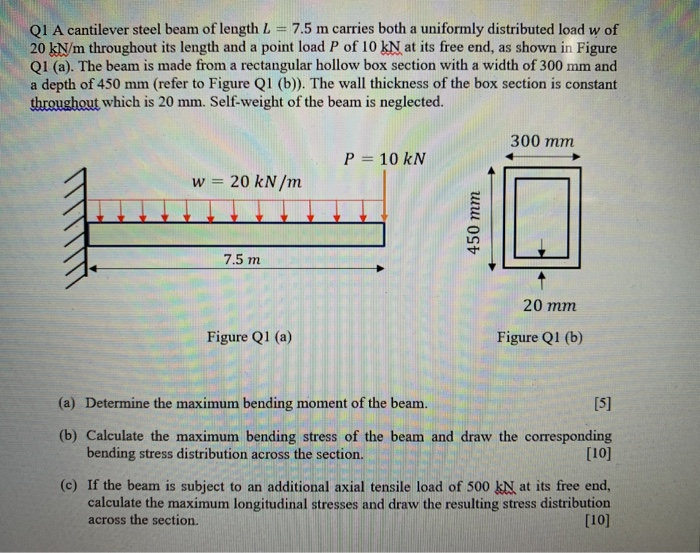QI A cantilever steel beam of length L 7.5 m carries both a uniformly distributed load w of 20 kN/m throughout its length and a point load P of 10 kN at its free end, as shown in Figure QI (a). The beam is made from a rectangular hollow box section with a width of 300 mm and a depth of 450 mm (refer to Figure Q1 (b)). The wall thickness of the box section is constant throughout which is...